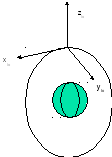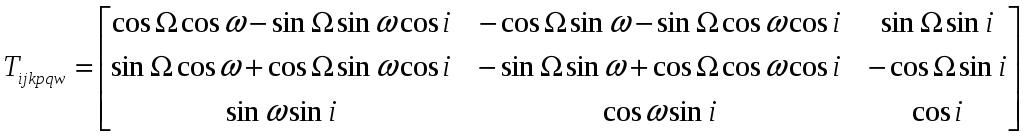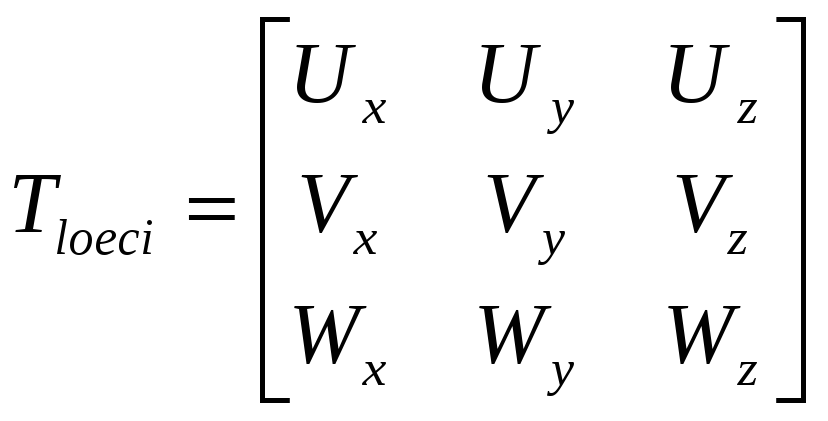# Spacecraft Attitude Determination Using Global Positioning Satellite SignalsFigure 3: Local orbital coordinate frame For this coordinate frame, x is defined as the direction of satellite motion, y the orbit normal, and z completes a right-handed coordinate frame. To rotate the position vectors into the local orbital frame, the vectors will first be rotated into the IJK coordinate frame using the following transformation matrix:(8) For whichis the right ascension of the ascending node,is the argument of perigee, andis the inclination (Sellers). The vector is then rotated into the local orbital coordinate frame using the following transformation matrix:(9) For whichDownload 1.81 Mb.Share with your friends: